The Art of Mathematics

# How to calculate

## 1. Syntax

Logicaly calculation is a set of expressions separated by semicolon. To successfully create or modify the file, follow three simple rules.

First. Write function calls like they are written in any book on mathematics, enclosing arguments into parentheses and separating expressions with semicolon:

``tanh(8.9);``

The only exception is Power function:

``3^2;``

Second. To engage current result use result symbol rslt:

``tanh(rslt);``

Third. To engage memory use store to memory and memory recall operators:

``tanh(8.9)−>mem[x];``
``tanh(mem[x]);``

Avoid using parentheses and brackets when naming values stored in memory.

## 2. Handbook

All the functions supported by calculator are present in Keypad. As well you can use the Keypad to access function handbook, which is the part of calculator Help.

There is Context Help button in the Toolbar — fig. 1.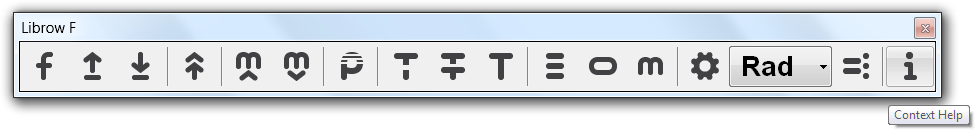Fig. 1. Context Help command in Toolbar.

Click the button — cursor will get a question mark attached to it — and now click the function of interest, for instance, ^ button — and Help will get you to Power function page of the hand-book. At your hand definition, plot and identities for any function.

## 3. Example

There are two ways you can enter expressions in calculator: you can use Keypad or directly type in from keyboard. For instance, enter

``sin(7.8)``

To calculate the current expression click = button or fire Ctrl+= shortcut — calculator evaluates it:

``sin(7.8)=0.13571557243430438``

Enter semicolon ; as separator and enter new expression:

``````sin(7.8)=0.13571557243430438;
rslt+cos(9)``````

There is an important symbol here: rslt, which represents the current result. Look at Status bar and in result pane you can see rslt = 0.13571557243430438 — the result of the last evaluation.

Now fire Ctrl+= shortcut and evaluate the last expression:

``````sin(7.8)=0.13571557243430438;
rslt+cos(9)=1.1234039130294422``````

Now change the first expression:

``````sin(7.8)+.4=0.13571557243430438;
rslt+cos(9)=1.1234039130294422``````

And click = all button or fire Ctrl+Shift+= shortcut. Both expressions get recalculated:

``````sin(7.8)+.4=0.53571557243430434;
rslt+cos(9)=1.5234039130294421``````

Now click clear button in Keypad to clear the editor and enter

``ln(0)``

Click = — the expression is not evaluated, but instead you have error message in Log window: Function has singularity at 0. There are other types of error and warnings you can get from calculator — details you can find in Log article.

## 4. Using memory

To address memory use mem operator and to push value to memory use operator — that's simple. Clear the editor and enter:

``4−>mem[frequency]``

Fire Ctrl+= to execute the command:

``4−>mem[frequency]=4``

Look at Memory window and you will find that cell frequency was allocated and value 4 was assigned to it. We have frequency, now add time:

``````4−>mem[frequency]=4;
3.5−>mem[time];``````

And finaly:

``````4−>mem[frequency]=4;
3.5−>mem[time];
sin(mem[time]*mem[frequency])``````

Fire Ctrl+Shift+= to calculate all expressions:

``````4−>mem[frequency]=4;
3.5−>mem[time]=3.5;
sin(mem[time]*mem[frequency])=0.24192189559966773``````

That is how you are addressing values put to memory.

You can clear the memory by clicking free button.

## 5. Calculations and datasets

Calculator supports two file types: .lcl which supposed to be calculation files and .lcm which supposed to be memory files. 'Supposed' because the difference between files is imaginary one. This difference allows you to separate processing and datasets. For instance, you can have several files that contain versions of the same data, like different sizes, and then you can have just one calculation file that intakes data and calculates square and volume. Or you can have several processing files, that process the same data different ways: first file calculates square and second one — volume.

So, you can save current editor content both as .lcl and .lcm file and you can load both types into editor back. You can Execute the .lcl file and you can Load the .lcm file. Both commands process file without loading into editor, and indeed both of them can process another type too. And as .lcl file .lcm file can contain any calculator command as well.

You can dump the current memory content as .lcm file.

Let us demonstrate this logic. Clear the editor window and enter:

``````4−>mem[a];
3−>mem[b];``````

Fire Ctrl+Shift+= to put values to memory and click Save memory button in Toolbar to save memory content as Rectangle 1.lcm.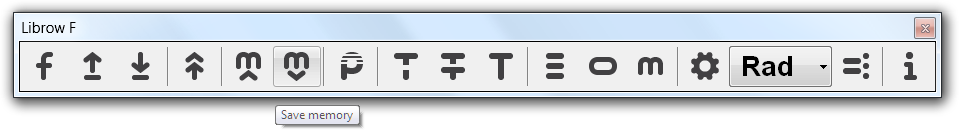Fig. 2. Memory Save command in Toolbar.

Now change the code to

``````5−>mem[a];
6−>mem[b];``````

and click File >> Save As to save the file as Rectangle 2.lcm — select the proper file type for that in File Save As dialog.Fig. 3. File Save As command in File menu.

Take into account, that first time you saved memory content, but this time you are saving the text you have entered in the editor — that is why it is not necessary to perform evaluation to put values into memory as before. Now clear the editor window and enter

``mem[a]*mem[b]``
which is the square of a×b rectangle. Now free the memory and click in Toolbar Load memory button to pick up the Rectangle 1.lcm file.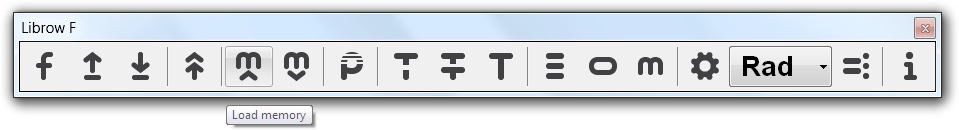Fig. 4. Memory Load command in Toolbar.

In Memory window you can see loaded values a and b. Fire Ctrl+= to perform calculation:

``mem[a]*mem[b]=12``

Now click Load memory again to load the Rectangle 2.lcm file. You can see in Memory window that a and b have changed their values. Fire Ctrl+= again to calculate new square:

``mem[a]*mem[b]=30``

Now let us inverse the order. Change the code

``mem[a]*mem[b]−>mem[square];``

and save it as Square.lcl file. Click Open button in Toolbar and open Rectangle 1.lcm.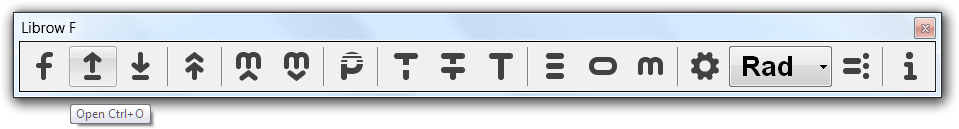Fig. 5. File Open command in Toolbar.

Evaluate the file to execute its memory commands and now click Execute button and pick up Square.lcl for execution.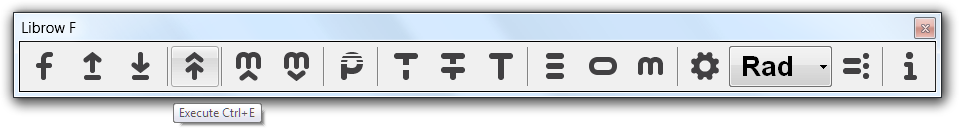Fig. 6. File Execute command in Toolbar.

Look at Memory — the execution resulted in memory value square, which keeps the square of a×b rectangle.